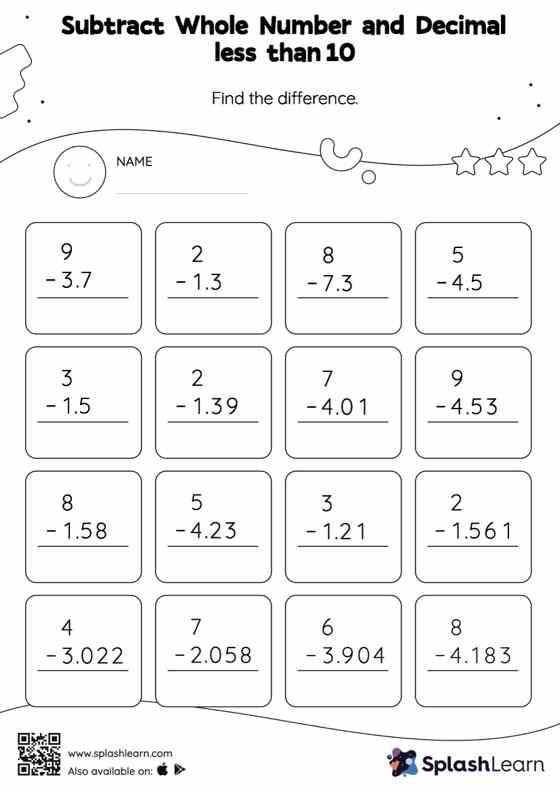# Subtract Whole Number and Decimal less than 10: Vertical Subtraction Worksheet

Home > Subtract Whole Number and Decimal less than 10: Vertical SubtractionMastery in decimals is now super easy! 5th graders can thoroughly practice in the subtract decimals with this worksheet.